# rfsystem

Perform circuit envelope simulation of RF system designed using `rfbudget`

## Description

Use the `rfsystem` System object™ to perform circuit envelope simulation of an RF system designed using an `rfbudget` object. You can use the `rfsystem` object to generate an RF Blockset™ model. This object supports vector inputs and has no frame-size limits.

To perform circuit envelope simulation of an RF system:

1. Create the `rfsystem` object and set its properties.

2. Call the object with arguments, as if it were a function.

## Creation

### Syntax

``rfs = rfsystem(rfb)``
``rfs = rfsystem(rfb,Name,Value)``

### Description

example

````rfs = rfsystem(rfb)` creates a System object from the RF system `rfb`. Use the System object, `rfs`, to perform circuit envelope simulation. The System object generates an untitled RF Blockset model of the RF system. Use Object Functions to open, save, close, or hide the RF Blockset model.```

example

````rfs = rfsystem(rfb,Name,Value)` sets Properties using name-value arguments. For example, `rfsystem(rfb,'ModelName','rfmodel')` sets the name of the RF Blockset model to `rfmodel`. ```

## Properties

expand all

Unless otherwise indicated, properties are nontunable, which means you cannot change their values after calling the object. Objects lock when you call them, and the `release` function unlocks them.

If a property is tunable, you can change its value at any time.

Name of the RF Blockset model, specified as a string scalar or character vector.

Example: `'ModelName','RFModel'`

Input frequency applied to each input of the RF system, specified as a non-negative scalar in Hz. This input frequency is derived from the RF system designed using an `rfbudget` object. Your RF system will have two inputs, I and Q, at DC if the `rfb.InputFrequency` is set to `0`.

Note

You can set `InputFrequency` to be a vector if you want to investigate the intermodulation of nearby signals going through the same architecture after construction of the` rfsystem` system object.

Output frequency to be computed at the output of the RF system, specified as a non-negative in Hz. This output frequency is derived from the RF system designed using an `rfbudget` object. Your RF system will have two outputs, I and Q, at DC if the value of the `rfb.OutputFrequency` is `0`.

Time step for circuit envelope simulation, specified as a positive scalar. This property sets the step size between simulations.

## Usage

### Syntax

``out = rfs(in)``

### Description

example

````out = rfs(in)` creates an RF Blockset circuit envelope simulation output `out` using input signal values `in`. Pass `in` as an input argument to an automatically-generated RF Blockset model. You can design four architectures, RF to RF, DC to RF, RF to DC, and DC to DC, using the `rfsystem` object. For more information, see Design RF-RF, IQ-RF, RF-IQ, and IQ-IQ Architectures. NotePassing multiple input vectors and concatenating the output vectors is equivalent to performing one long simulation with a vertically-concatenated input. ```

### Input Arguments

expand all

Input time-domain signal, specified as column vectors. Specify `in` as an array of column vectors when there are N `'InputFrequency'` values.

### Output Arguments

expand all

Output time-domain signal, returned as column vectors. `out` is returned as an array of column vectors when there are N `'OutputFrequency'` values to be computed.

## Object Functions

To use an object function, specify the System object™ as the first input argument. For example, to release system resources of a System object named `obj`, use this syntax:

`release(obj)`

expand all

 `open_system` Open RF Blockset model created using `rfsystem` `save_system` Save RF Blockset model created using `rfsystem` `close_system` Close RF Blockset model window created using `rfsystem` `hide_system` Hide RF Blockset model window created using `rfsystem`

expand all

 `step` Run System object algorithm `release` Release resources and allow changes to System object property values and input characteristics `reset` Reset internal states of System object

## Examples

collapse all

Design an RF receiver to perform circuit envelope simulation.

Create fifth- and seventh-order bandpass RF filters.

```f1 = rffilter('ResponseType','Bandpass','FilterOrder',5, ... 'PassbandFrequency',[4.85 5.15]*1e9); f2 = rffilter('ResponseType','Bandpass','FilterOrder',7, ... 'PassbandFrequency',[10 130]*1e6); ```

Create two amplifier objects with `3` dB and `5` dB gain, respectively.

```a1 = amplifier('Gain',3,'NF',1.53,'OIP3',35); a2 = amplifier('Gain',5,'NF',8,'OIP3',37); ```

Create a modulator with a local frequency of `4.93` GHz.

```d = modulator('Gain',0,'NF',4,'OIP3',50,'LO',4.93e9, ... 'ConverterType','Down'); ```

Design an RF receiver with the budget elements at an input frequency of `5` GHz, an available input power of `-30` dBm, and a bandwidth of `200` MHz.

```rfb = rfbudget([f1 a1 d f2 a2],5e9,-30,200e6); ```

Create an RF system for the RF receiver using the `rfbudget` object.

```rfs = rfsystem(rfb); ```

Specify input time-domain signal for the RF system.

```in = [1e-3*ones(8,1); zeros(8,1)] .* ones(1,10); in = in(:); ```

Calculate the output time-domain signal of the RF system.

```out = rfs(in); out = [out; rfs(in)]; ```

Specify the sample time of the RF system.

```t = rfs.SampleTime*(0:length(out)-1); ```

Plot the simulated output.

```plot(t,[in; in],'-o',t,abs(out),'-+') grid on ```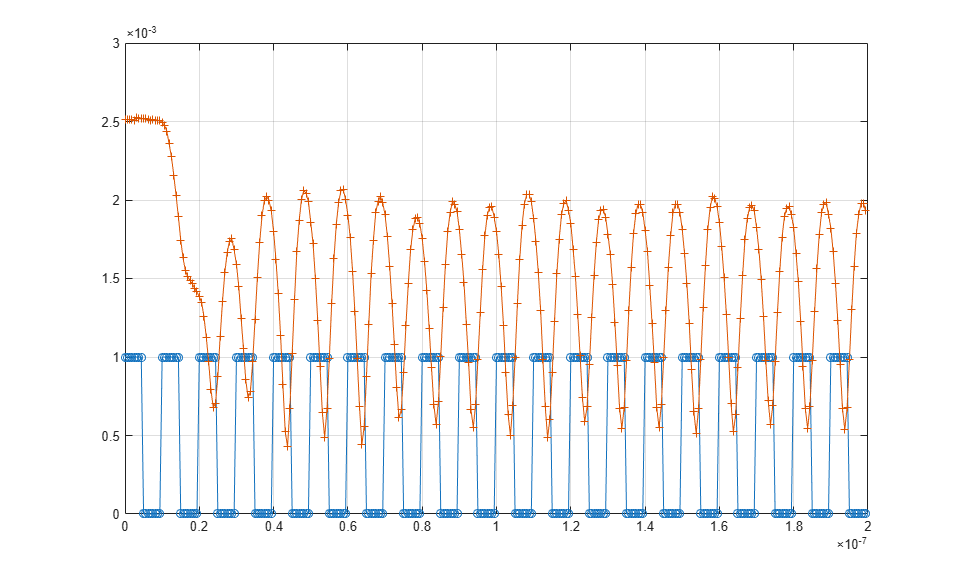Release system resources and turn off fast restart.

```release(rfs) ```

Open an RF Blockset model of the designed RF system using the `open_system` object function.

```open_system(rfs) ```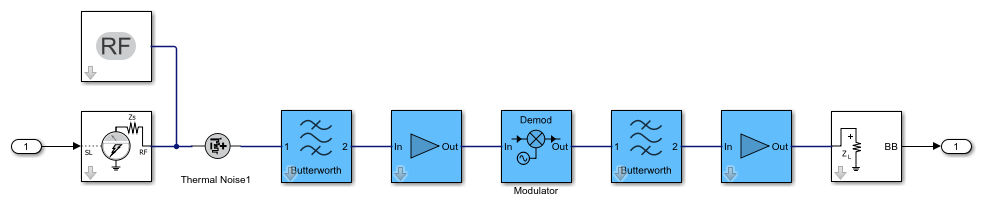Design four different chain architectures using an RF System object.

Create an input column vector.

```in = (1:8)'; ```

Design RF-RF Architecture

Create an `rfbudget` object using an `amplifier` object.

```a = amplifier; ```

Calculate the RF budget of the amplifier at an input frequency of `5` GHz, an available input power of `–30` dBm, and a bandwidth of `10` KHz.

```rfb = rfbudget(a,5e9,-30,10e3); ```

Create an RF system using the `rfbudget` object.

```rfs = rfsystem(rfb); ```

Create an RF-RF architecture using the input column vector.

```out0 = rfs(in); ```

Release system resources and turn off fast restart.

```release(rfs) ```

Open an RF Blockset model of the RF system.

```open_system(rfs) ```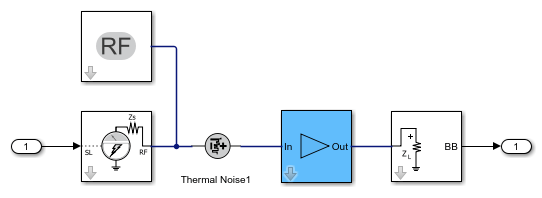Design IQ-RF Architecture

Use a `modulator` object with an up converter to create an `rfbudget` object.

```u = modulator('ConverterType','Up','LO',1e9); ```

Calculate the RF budget of the modulator at an input frequency of `0` GHz, an available input power of `–30` dBm, and a bandwidth of `10` KHz.

```rfb2 = rfbudget(u,0,-30,10e3); ```

Create an RF system using the `rfbudget` object.

```rfs2 = rfsystem(rfb2); ```

Create an IQ-RF architecture using the input column vector.

```inI = in; inQ = in; out = rfs2(inI,inQ); ```

Release system resources and turn off fast restart.

```release(rfs2) ```

Open an RF Blockset model of the RF system.

```open_system(rfs2) ```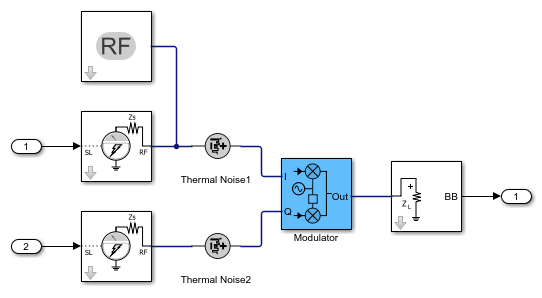Design RF-IQ Architecture

Use a `modulator` object with a down converter to create an `rfbudget` object.

```d = modulator('ConverterType','Down','LO',1e9); ```

Calculate the RF budget of the modulator at an input frequency of `1` GHz, an available input power of `–30` dBm, and a bandwidth of `10` KHz.

```rfb3 = rfbudget(d,1e9,-30,10e3); ```

Create an RF system using the `rfbudget` object.

```rfs3 = rfsystem(rfb3); ```

Create an RF-IQ architecture using the input column vector.

```[outI,outQ] = rfs3(in); ```

Release system resources and turn off fast restart.

```release(rfs3) ```

Open an RF Blockset model of the RF system.

```open_system(rfs3) ```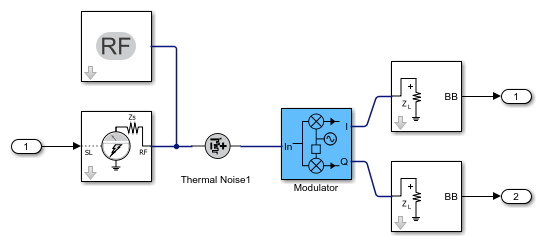Design IQ-IQ Architecture

Create an `rfbudget` object using an `amplifier` object.

```a1 = amplifier; ```

Calculate the RF budget of the amplifier at an input frequency of `0` GHz, an available input power of `–30` dBm, and a bandwidth of `10` KHz.

```rfb4 = rfbudget(a1,0,-30,10e3); ```

Create an RF system using the `rfbudget` object.

```rfs4 = rfsystem(rfb4); ```

Create an IQ-IQ architecture using the input column vector.

```[outI2,outQ2] = rfs4(inI,inQ); ```

Release system resources and turn off fast restart.

```release(rfs4) ```

Open an RF Blockset model of the RF system.

```open_system(rfs4) ```Create a fifth-order bandpass RF filter.

```f1 = rffilter('ResponseType','Bandpass','FilterOrder',5,'PassbandFrequency',[4.85 5.15]*1e9); ```

Create an amplifier with the gain of `3` dB, noise figure of `1.53` dB, and OIP3 of `35` dBm.

```a1 = amplifier('Gain',3,'NF',1.53,'OIP3',35); ```

Create an `rfbudget` object using these elements at an input frequency of `5` GHz, an available input power of `-30` dBm, and a bandwidth of `200` MHz.

```rfb = rfbudget([f1 a1],5e9,-30,200e6); ```

Create an RF system using the rfbdget object. Name the model and save the RF Blockset model.

```rfs = rfsystem(rfb,'ModelName','myRFSystem_Model') save_system(rfs); ```
```rfs = rfsystem with properties: ModelName: 'myRFSystem_Model' SampleTime: 6.2500e-10 InputFrequency: 5.0000e+09 OutputFrequency: 5.0000e+09 ```